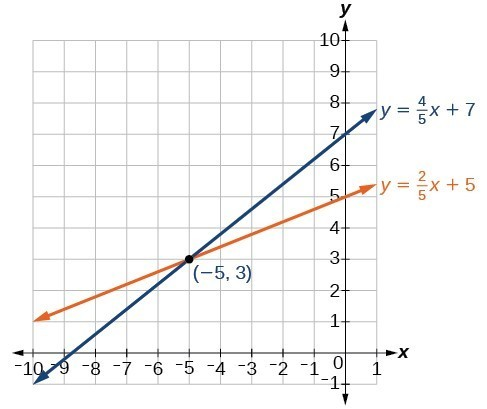Solutions to Try Its

1. Not a solution.

2. The solution to the system is the ordered pair $\left(-5,3\right)$.3. $\left(-2,-5\right)$

4. $\left(-6,-2\right)$

5. $\left(10,-4\right)$

6. No solution. It is an inconsistent system.

7. The system is dependent so there are infinite solutions of the form $\left(x,2x+5\right)$.

Solutions to Odd-Numbered Exercises

1. No, you can either have zero, one, or infinitely many. Examine graphs.

3. This means there is no realistic break-even point. By the time the company produces one unit they are already making profit.

5. You can solve by substitution (isolating $x$ or $y$ ), graphically, or by addition.

7. Yes

9. Yes

11. $\left(-1,2\right)$

13. $\left(-3,1\right)$

15. $\left(-\frac{3}{5},0\right)$

17. No solutions exist.

19. $\left(\frac{72}{5},\frac{132}{5}\right)$

21. $\left(6,-6\right)$

23. $\left(-\frac{1}{2},\frac{1}{10}\right)$

25. No solutions exist.

27. $\left(-\frac{1}{5},\frac{2}{3}\right)$

29. $\left(x,\frac{x+3}{2}\right)$

31. $\left(-4,4\right)$

33. $\left(\frac{1}{2},\frac{1}{8}\right)$

35. $\left(\frac{1}{6},0\right)$

37. $\left(x,2\left(7x - 6\right)\right)$

39. $\left(-\frac{5}{6},\frac{4}{3}\right)$

41. Consistent with one solution

43. Consistent with one solution

45. Dependent with infinitely many solutions

47. $\left(-3.08,4.91\right)$

49. $\left(-1.52,2.29\right)$

51. $\left(\frac{A+B}{2},\frac{A-B}{2}\right)$

53. $\left(\frac{-1}{A-B},\frac{A}{A-B}\right)$

55. $\left(\frac{CE-BF}{BD-AE},\frac{AF-CD}{BD-AE}\right)$

57. They never turn a profit.

59. $\left(1,250,100,000\right)$

61. The numbers are 7.5 and 20.5.

63. 24,000

65. 790 sophomores, 805 freshman

67. 56 men, 74 women

69. 10 gallons of 10% solution, 15 gallons of 60% solution

71. Swan Peak: $750,000, Riverside:$350,000

73. $12,500 in the first account,$10,500 in the second account.

75. High-tops: 45, Low-tops: 15